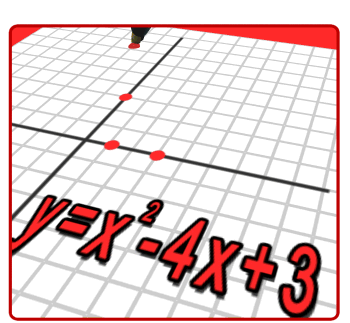• Welcome to Mr. deWolfe's Mathematics site.  Below you will find information about Frenchtown's mathematics courses for grades 6-8.

PARCC TESTING: May, 2020 (dates TBD)I.         Ratios and Proportional Relationships

a.   Chapter 1 - Ratios and Rates

b.   Chapter 2 - Fractions, Decimals, and Percents

II.               Number Sense

a.   Chapter 3 - Compute with Multi-Digit Numbers

b.     Chapter 4 – Multiply and Divide Fractions

i.     Multiplying and dividing with whole numbers, fractions, and mixed numbers

c.     Chapter 5 – Integers and the Coordinate Plane

i.     Integers and graphing, absolute value, comparing and ordering integers and rational numbers, terminating and repeating decimals, the coordinate plane

III.             Expressions and Equations

a.     Chapter 6 – Expressions

i.     Powers, exponents, expressions, variables and expressions, properties, distributive property

b.     Chapter 7 – Equations

i.     Solving Equations with addition, subtraction, multiplication and division

c.      Chapter 8 – Functions and Inequalities

i.     Funtion tables, function rules, multiple representation of functions, writing and graphing inequalities, solving one step inequalities

IV.           Geometry

a.     Chapter 9 – Area

i.     Area of parallelograms, area triangles, area of trapezoids, polygons on the coordinate plane, area of irregular figures

b.     Chapter 10 – Volume and Surface Area

i.     Volume and surface area of rectangular and triangular prisms; surface area of pyramids

V.            Statistics and Probability

a.     Chapter 11 – Statistical Measures

i.     Mean, median, mode, measures of variation

b.     Chapter 12 – Statistical Displays

i.     Line plots, histograms, box plots, data distributions

Classwork: 5-10 points

Homework: 5-20 points

Quizzes: 50 points

Tests/Projects: 30-100 pointsI.               Number System Unit

a.   Chapter 1 - The Language of Algebra

b.   Chapter 2 - Operations with Integers

c.     Chapter 3 – Integers

i.     Integers, absolute value, operations with integers

d.     Chapter 4 – Rational Numbers

i.     Terminating and repeating decimals, comparing and ordering rational numbers, adding and subtracting like and unlike fractions, multiplying and dividing fractions, converting between measurement systems

II.             Expressions and Equations Unit

a.     Chapter 5 – Expressions

i.     Algebraic expressions, sequences, properties of operations, distributive property, adding subtracting and factoring linear expressions

b.     Chapter 6 – Equations and Inequalities

i.     Solving one-step equations with addition and subtracting, one-step equations with multiplication and division, two-step equations, solving inequalities, solving two-step inequalities

III.           Geometry Unit

a.     Chapter 7 – Geometric Figures

i.     Complementary and supplementary angles, creating triangles, scale drawings, cross sections

b.     Chapter 8 – Measure Figures

i.     Circumference, area, surface area, and nets of circles, prisms, pyramids, and composite figures

IV.            Statistics and Probability Unit

a.     Chapter 9 - Probability

i.     Theoretical and experimental probability, compound events, independent and dependent events, permutation

b.     Chapter 10 – Statistics

i.     Comparing populations, making predictions, biased and unbiased samples, graphs and statistics, selecting appropriate displays

Classwork: 5-10 points

Homework: 5-20 points

Quizzes: 50 points

Tests/Projects: 30-100 pointsI.         Ratios and Proportional Relationships

a.    Chapter 1 - Ratios and Proportional Reasoning

b.    Chapter 2 - Percents

II.        The Number System

a.    Chapter 3 - Integers

b.    Chapter 4 - Rational Numbers

III.       Expressions and Equations

a.    Chapter 5 - Expressions

b.    Chapter 6 - Equations and Inequalities

IV.      Geometry

a.    Chapter 7 - Geometric Figures

b.    Chapter 8 - Measure Figures

V.      Statistics and Probability

a.    Chapter 9 - Probability

b.    Chapter 10 - Statistics

Classwork:  5-10 points

Homework:  5-20 points

Quizzes: 50 points

Tests/Projects: 30-100 pointsI.         The Numbers System

a.    Chapter 1 - Real Numbers

II.       Expressions and Equations

a.    Chapter 2 - Equations in One Variable

b.    Chapter 3 - Equations in Two Variables

i.     Slope, Rate of Change, y=mx form, slope intercept form, slope triangles, writing linear equations, solving systems of equations by graphing and algebraically

III.      Functions

a.     Chapter 4 – Functions

i.     Representing relationships, relations, linear functions, comparing properties of functions, nonlinear functions, quadratic functions

IV.           Geometry

a.     Chapter 5 – Triangles

i.     Lines, geometric proofs, angles of triangles, polygons and angles, right triangle relationships, Pythagorean theorem,  distance on the coordinate plane

b.     Chapter 6 – Transformations

i.     Translations, reflections, rotational symmetry, rotations, dilations

c.      Chapter 7 – Congruence and Similarity

i.     Congruence and transformations, congruent triangles, similarity, similar polygons, area and perimeter of similar figures

d.     Chapter 8 – Volume and Surface Area

i.     Volume and surface area of cylinders, cones, and spheres

V.            Statistics and Probability

a.     Chapter 9 – Statistics and Probability

i.     Measures of central tendency, box plots, scatter plots, histograms,

Classwork: 5-10 points

Homework: 5-20 points

Quizzes: 50 points

Tests/Projects: 20-100 points